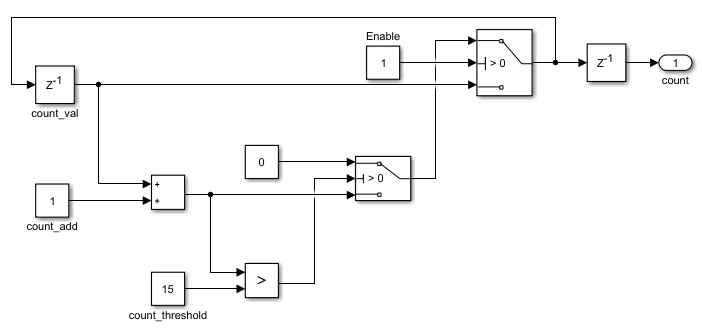## Model a Counter for HDL Code Generation

This design pattern shows a MATLAB® example of a counter, which is suitable for HDL code generation.

### MATLAB Counter

This design pattern demonstrates two best practices for writing MATLAB code for HDL code generation:

• Initialize persistent variables to a specific value. In this example, an `if` statement and the `isempty ` function initialize the persistent variable. If the persistent variable is not initialized then HDL code cannot be generated.

• Inside a function, read persistent variables before they are modified, in order for the persistent variables to be inferred as registers.

This Simulink® model illustrates the counter modeled in this example.To learn how to model the counter in Simulink, see Create HDL-Compatible Simulink Model.

### MATLAB Code for the Counter

The function `mlhdlc_counter` is a behavioral model of a four bit synchronous up counter. The input signal, `enable_ctr`, triggers the value of the count register, `count_val`, to increase by one. The counter continues to increase by one each time the input is nonzero, until the count reaches a limit of 15. After the counter reaches this limit, the counter returns to zero. A persistent variable, which is initialized to zero, represents the current value of the count. Two `if` statements determine the value of the count based on the input.

The following section of code defines the `mldhlc_counter` function.

```%#codegen function count = mlhdlc_counter(enable_ctr) %four bit synchronous up counter %persistent variable for the state persistent count_val; if isempty(count_val) count_val = 0; end %counting up if enable_ctr count_val=count_val+1; %limit to four bits if count_val>15 count_val=0; end end count=count_val; end ```